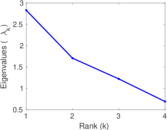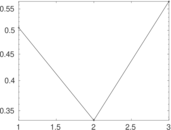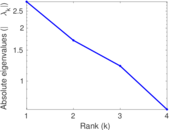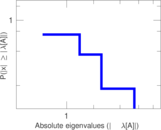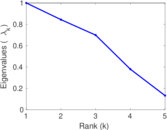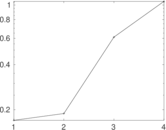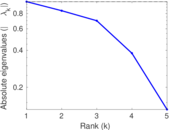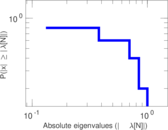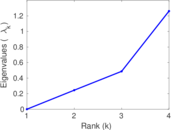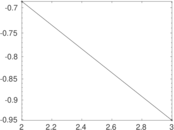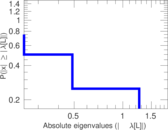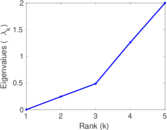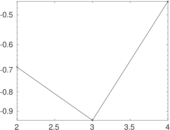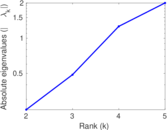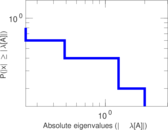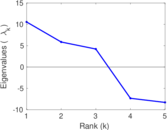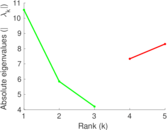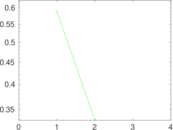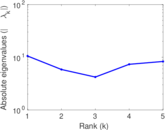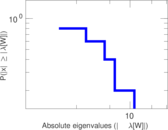# South African companies

This bipartite network contains person–company shared leadership relations of "the five most representative companies" that are claimed to represent "the small inner ring of South African Finance". Left nodes represent persons and right nodes represent companies. An edge between a person and a company shows that the person had a leadership position in that company.

 Code `SC` Internal name `brunson_south-africa` Name South African companies Data source https://github.com/corybrunson/triadic AvailabilityDataset is available for download Consistency checkDataset passed all tests Category Affiliation network Node meaning Person, company Edge meaning Leadership Network formatBipartite, undirected Edge typeUnweighted, no multiple edges

## Statistics

 Size n = 11 Left size n1 = 6 Right size n2 = 5 Volume m = 13 Wedge count s = 13 Claw count z = 10 Cross count x = 2 Square count q = 4 4-Tour count T4 = 150 Maximum degree dmax = 4 Maximum left degree d1max = 3 Maximum right degree d2max = 4 Average degree d = 2.363 64 Average left degree d1 = 2.166 67 Average right degree d2 = 2.600 00 Fill p = 0.433 333 Size of LCC N = 2 Diameter δ = 4 50-Percentile effective diameter δ0.5 = 2.000 00 90-Percentile effective diameter δ0.9 = 0.700 000 Median distance δM = 0 Mean distance δm = 0.333 333 Gini coefficient G = 0.230 769 Balanced inequality ratio P = 0.307 692 Left balanced inequality ratio P1 = 0.461 538 Right balanced inequality ratio P2 = 0.384 615 Relative edge distribution entropy Her = 0.963 938 Power law exponent γ = 2.304 01 Tail power law exponent γt = 3.211 00 Tail power law exponent with p γ3 = 3.211 00 p-value p = 0.376 000 Left tail power law exponent with p γ3,1 = 5.331 00 Left p-value p1 = 0.549 000 Right tail power law exponent with p γ3,2 = 1.811 00 Right p-value p2 = 0.029 000 0 Degree assortativity ρ = +0.184 466 Degree assortativity p-value pρ = 0.546 309 Spectral norm α = 2.829 33 Algebraic connectivity a = 0.244 822 Spectral separation |λ1[A] / λ2[A]| = 1.658 85 Controllability C = 1 Relative controllability Cr = 0.090 909 1

## Plots

### Fruchterman–Reingold graph drawing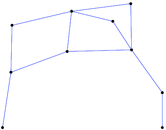### Degree distribution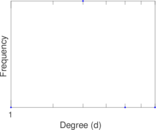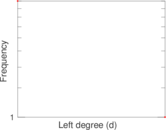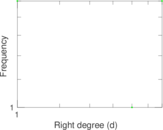### Cumulative degree distribution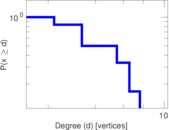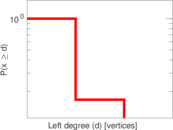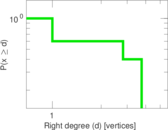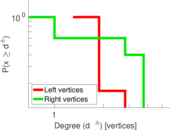### Lorenz curve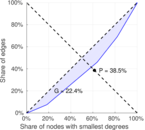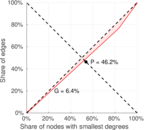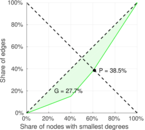### Spectral distribution of the adjacency matrix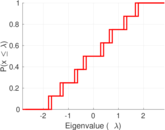### Spectral distribution of the normalized adjacency matrix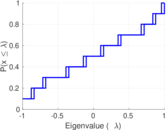### Spectral distribution of the Laplacian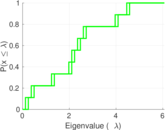### Spectral graph drawing based on the adjacency matrix### Spectral graph drawing based on the Laplacian### Spectral graph drawing based on the normalized adjacency matrix### Degree assortativity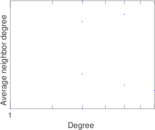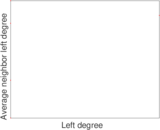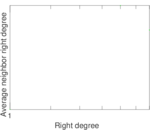### Zipf plot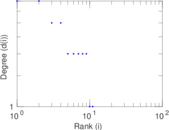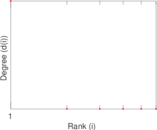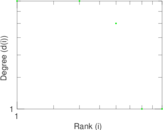### Hop distribution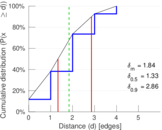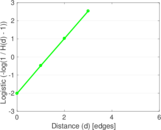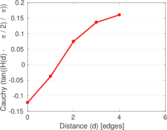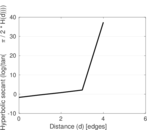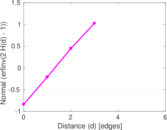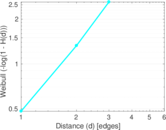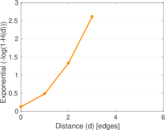### Double Laplacian graph drawing### Delaunay graph drawing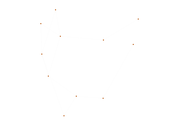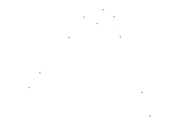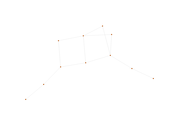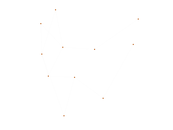### Matrix decompositions plots